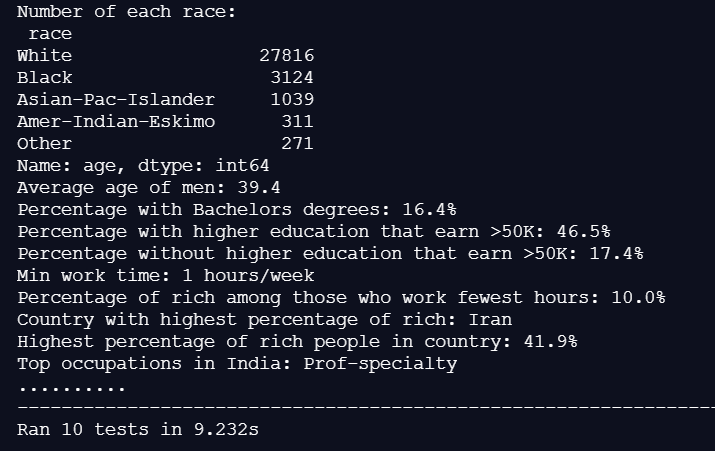signed

QiShunwang

“诚信为本、客户至上”

# Medical Data Visualizer

2021/4/26 23:11:07   来源：

• Introduction
• Code
• DataSet
• Result
• Last
• End

# Introduction

In this challenge you must analyze demographic data using Pandas. You are given dataset of demographic data that was extracted from the 1994 Census database.

FreeCodeCamp

### Code

``````import pandas as pd

def calculate_demographic_data(print_data=True):
# Read data from file
df = pd.read_csv('adult.data.csv')

# How many of each race are represented in this dataset? This should be a Pandas series with race names as the index labels.
race_count = df.groupby("race").count()['age'].sort_values(ascending=False)

# What is the average age of men?
average_age_men = round(df[df['sex'] == 'Male']['age'].mean(), 1)

# What is the percentage of people who have a Bachelor's degree?
percentage_bachelors = round(df[df['education'] == 'Bachelors']['education'].count() /df['education'].count() * 100, 1)

# What percentage of people with advanced education (`Bachelors`, `Masters`, or `Doctorate`) make more than 50K?
# What percentage of people without advanced education make more than 50K?

# with and without `Bachelors`, `Masters`, or `Doctorate`
higher_education = df[((df['education'] == 'Bachelors') | (df['education'] == 'Masters') | (df['education'] == 'Doctorate'))]['education'].count()
lower_education = df[((df['education'] != 'Bachelors') & (df['education'] != 'Masters') & (df['education'] != 'Doctorate'))]['education'].count()

# percentage with salary >50K
higher_education_rich = round(df[(df['salary'] == '>50K') & ((df['education'] == 'Bachelors') | (df['education'] == 'Masters') | (df['education'] == 'Doctorate'))]['salary'].count() / higher_education * 100, 1)

lower_education_rich = round(df[(df['salary'] == '>50K') & ((df['education'] != 'Bachelors') & (df['education'] != 'Masters') & (df['education'] != 'Doctorate'))]['education'].count() / lower_education * 100, 1)

# What is the minimum number of hours a person works per week (hours-per-week feature)?
min_work_hours = df['hours-per-week'].min()

# What percentage of the people who work the minimum number of hours per week have a salary of >50K?
num_min_workers = df[(df['hours-per-week'] == min_work_hours) & (df['salary'] == '>50K')]['salary'].count()

rich_percentage = round(num_min_workers / df[(df['hours-per-week'] == min_work_hours)]['hours-per-week'].count() * 100, 1)

# What country has the highest percentage of people that earn >50K?
# reference resources：https://www.reddit.com/r/FreeCodeCamp/comments/le7ynx/data_analysis_with_python_projects_solving/
salary = df.loc[df['salary'] == '>50K']['native-country'].value_counts()
population = df['native-country'].value_counts()
highest_earning_country = (salary / population).sort_values(ascending=False).index
highest_earning_country_percentage = round((salary / population * 100).max(), 1)

# Identify the most popular occupation for those who earn >50K in India.
top_IN_occupation = df[(df['salary'] == '>50K') & (df['native-country'] == 'India')]['occupation'].mode()
# print(top_IN_occupation)

# DO NOT MODIFY BELOW THIS LINE

if print_data:
print("Number of each race:\n", race_count)
print("Average age of men:", average_age_men)
print(f"Percentage with Bachelors degrees: {percentage_bachelors}%")
print(f"Percentage with higher education that earn >50K: {higher_education_rich}%")
print(f"Percentage without higher education that earn >50K: {lower_education_rich}%")
print(f"Min work time: {min_work_hours} hours/week")
print(f"Percentage of rich among those who work fewest hours: {rich_percentage}%")
print("Country with highest percentage of rich:", highest_earning_country)
print(f"Highest percentage of rich people in country: {highest_earning_country_percentage}%")
print("Top occupations in India:", top_IN_occupation)

return {
'race_count': race_count,
'average_age_men': average_age_men,
'percentage_bachelors': percentage_bachelors,
'higher_education_rich': higher_education_rich,
'lower_education_rich': lower_education_rich,
'min_work_hours': min_work_hours,
'rich_percentage': rich_percentage,
'highest_earning_country': highest_earning_country,
'highest_earning_country_percentage':
highest_earning_country_percentage,
'top_IN_occupation': top_IN_occupation
}

``````

adult.data.csv

### Result### Last

1. 前几个问题自然而然的想到了分组统计，所以就用了groupby和count。根据groupby分组然后用count统计。
2. pandas的排序用的是sort_values,有values自然就会有其他的，不然直接用sort关键字作方法就可以了。这里的另外一种排序是sort_index根据索引排序。正倒序的关键参数也和Python的不一样，这里的是Boolean类型的ascending翻译过来就是上升、升序，那逆序就是False了。
3. 这里对列的筛选用的最多的是嵌套的dataframe：`df[df['columns'] OPERATOR term]` 多个筛选条件的时候用的是`& |`分别对于Python的`and or`。另外一种筛选方法是使用loc，其实跟第一种方式区别不大:`df[(df['salary'] == '>50K')], df.loc[df['salary'] == '>50K']`结果都一样。
4. 到最后两三个问题的时候，知识就不够用了。后面看了一个视频才知道用到了没见过的方法`value_counts``mode``value_counts`是对值进行统计，有点像分组统计的味道，而`mode`则是求Series的众数。
5. round是四舍五入保留小数位的函数。

### End